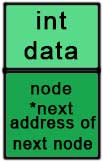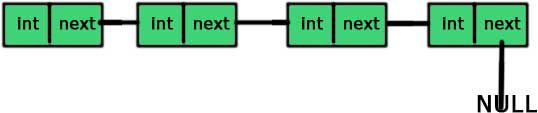May 21, 2017 147144

Linked list is one of the most important data structures. We often face situations, where the data is dynamic in nature and number of data can’t be predicted or the number of data keeps changing during program execution. Linked lists are very useful in this type of situations.

The implementation of a linked list in C is done using pointers. You can go through the pointers chapter if you don’t have a strong grip over it. You can also practice a good number of questions from practice section.

A linked list is made up of many nodes which are connected in nature. Every node is mainly divided into two parts, one part holds the data and the other part is connected to a different node. It is similar to the picture given below.Here, each node contains a data member (the upper part of the picture) and link to another node(lower part of the picture).

Notice that the last node doesn’t point to any other node and just stores NULL.

In C, we achieve this functionality by using structures and pointers. Each structure represents a node having some data and also a pointer to another structure of the same kind. This pointer holds the address of the next node and creates the link between two nodes. So, the structure is something like:

struct node
{
int data;
struct node *next;
};


The first data member of the structure (named node) is an integer to hold an integer and the second data member is the pointer to a node (same structure). This means that the second data member holds the address of the next node and in this way, every node is connected as represented in the picture above.

The picture representing the above structure is given below.And the picture representing the linked list is:So, if we have access to the first node then we can access any node of the linked list. For example, if ‘a’ is a node then a->next is the node next to the ‘a’ (the pointer storing the address of the next node is named ‘next’).

One thing you should notice here is that we can easily access the next node but there is no way of accessing the previous node and this is the limitation of singly linked list.

## Coding up a linked list

You are now clear with the concepts of a linked list. Let’s code it up. The first part is to create a node (structure).

#include <stdio.h>
#include <stdlib.h>

struct node
{
int data;
struct node *next;
};


The second and the most important part of a linked list is to always keep the track of the first node because access to the first node means access to the entire list. So, let’s call our first node as ‘ head’.

#include <stdio.h>
#include <stdlib.h>

struct node
{
int data;
struct node *next;
};

int main()
{
return 0;
}


We have made three nodes – head, prev and p. You will see the function of prev in the explanation of the next block of code.

Now, let’s create a node ‘p’.

#include <stdio.h>
#include <stdlib.h>

struct node
{
int data;
struct node *next;
};

int main()
{
p=malloc(sizeof(struct node));
scanf("%d",&p->data);
p->next=NULL;
return 0;
}


If you are not familiar with the ‘malloc’ function, then just read the dynamic memory allocation chapter.

p=malloc(sizeof(struct node)) – We are allocating the space required for a node by the malloc function. Now, ‘p’ points to a node (or space allocated for the node).

scanf("%d",&p->data) –  We are giving a value to the ‘data’ of ‘p’ after taking the input from the user.

p->next=NULL – We have given the value to ‘data’ in the previous line and a value of the pointer ‘next’ (NULL) in this line and thus making our node ‘p’ complete.

Let’s create our linked list by joining the nodes.

#include <stdio.h>
#include <stdlib.h>

struct node
{
int data;
struct node *next;
};

int main()
{
int n,i;
printf ("number of elements:");
scanf("%d",&n);
for(i=0;i<n;i++)
{
p=malloc(sizeof(struct node));
scanf("%d",&p->data);
p->next=NULL;
else
prev->next=p;
prev=p;
}
return 0;
}


We are storing n number of elements in our linked list.

if(head==NULL) – If the ‘head’ is NULL, then our linked list is not created.

head=p – We have given the value to the ‘head’ and thus made the first node of our linked list.

else – The linked list is already there and we just have to add a node in this linked list.

prev->next=p – We have used the prev to store the record of the previous node to the current node (the last node from the previous iteration) (you will see in the next line). The ‘next’ of this prev is holding NULL till now. We pointed it to the node ‘p’ and hence added a node to our linked list.

prev=p – We made the last node ‘prev’ for the next iteration.

Try to understand the code by allocating two to three nodes by above mechanism and you will get it.

### Next:

Liked the post?
Developer and founder of CodesDope.